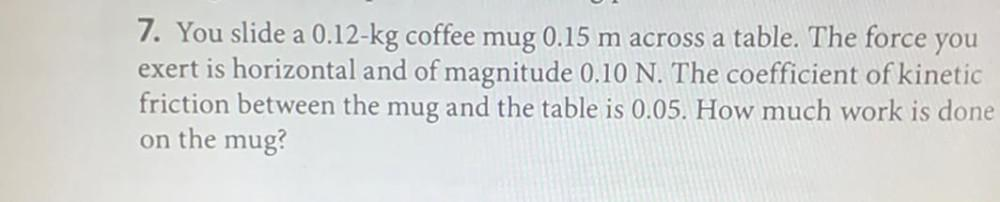Question:

# You slide a 0.12-kg coffee mug 0.15 m across a table. The force you exert is horizontal and of magnitude 0.10 N. The coefficient of kinetic friction between the mug and the table is 0.05. How much worYou slide a 0.12-kg coffee mug 0.15 m across a table. The force you exert is horizontal and of magnitude 0.10 N. The coefficient of kinetic friction between the mug and the table is 0.05. How much work is done on the mug?